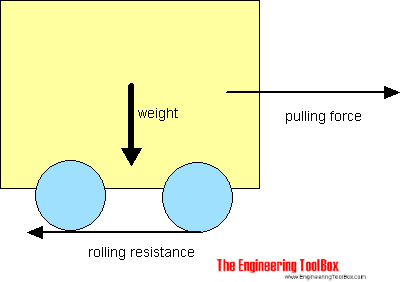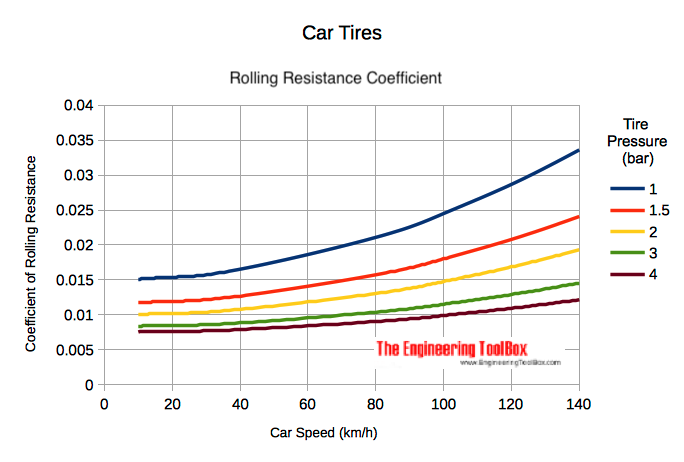Engineering ToolBox - Resources, Tools and Basic Information for Engineering and Design of Technical Applications!

# Rolling Resistance

## Rolling friction and rolling resistance.

The force that resists the motion of a body rolling on a surface is called the rolling resistance or the rolling friction.The rolling resistance can be expressed by the generic equation

Fr = c W                                   (1)

where

Fr = rolling resistance or rolling friction (N, lbf)

c = rolling resistance coefficient - dimensionless (coefficient of rolling friction - CRF)

W = m ag

= normal force - or weight - of the body (N, lbf)

m = mass of body (kg, lb)

ag = acceleration of gravity (9.81 m/s2, 32.174 ft/s2)

Note that the rolling resistance coefficient - c - is influenced by different variables like wheel design, rolling surface, wheel dimensions and more.

The rolling resistance can alternatively be expressed as

Fr = cl W / r                                (2)

where

cl = rolling resistance coefficient - dimension length (coefficient of rolling friction) (mm, in)

r = radius of wheel (mm, in)

### Rolling Friction Coefficients

Some typical rolling coefficients:

Rolling Friction Coefficients
Rolling Resistance Coefficient
ccl (mm)
0.001 - 0.002 0.5 railroad steel wheels on steel rails
0.001 bicycle tire on wooden track
0.002 - 0.005 low resistance tubeless tires
0.002 bicycle tire on concrete
0.004 bicycle tire on asphalt road
0.005 dirty tram rails
0.006 - 0.01 truck tire on asphalt
0.008 bicycle tire on rough paved road
0.01 - 0.015 ordinary car tires on concrete, new asphalt, cobbles small new
0.02 car tires on tar or asphalt
0.02 car tires on gravel - rolled new
0.03 car tires on cobbles  - large worn
0.04 - 0.08 car tire on solid sand, gravel loose worn, soil medium hard
0.2 - 0.4 car tire on loose sand

### Rolling Coefficients Cars

The rolling coefficients for air filled tires on dry roads can be estimated

c = 0.005 + (1 / p) (0.01 + 0.0095 (v / 100)2)                     (3)

where

c = rolling coefficient

p = tire pressure (bar)

v = velocity (km/h)

#### Example - Wheel Pressure & Rolling Resistance Coefficient

The standard wheel pressure in a Tesla Model 3 is 2.9 bar (42 psi). The rolling friction coefficient at 90 km/h (56 mph) can be calculated from (3) as

c = 0.005 + (1 / (2.9 bar)) (0.01 + 0.0095 ((90 km/h) / 100)2)

= 0.011

Increasing the pressure to 3.5 bar reduces the rolling resitance coefficient to

c = 0.005 + (1 / (3.5 bar)) (0.01 + 0.0095 ((90 km/h) / 100)2)

= 0.010

- or

((0.011 - 0.10) / 0.011) 100% = 9%

###• 1 bar = 105 Pa = 14.5 psi
• 1 km/h = 0.6214 mph

### Example - The Rolling Resistance of a Car on Asphalt

The rolling resistance for all four wheels in a car with total weight 1500 kg on asphalt with rolling friction coefficient 0.03 can be estimated with the generic equation 1 as

Fr = 0.03 (1500 kg) (9.81 m/s2)

= 441 N

= 0.44 kN

The rolling resistance for one wheel can be calculated as

Fr = 0.03 (1500 kg / 4) (9.81 m/s2)

= 110 N

= 0.11 kN

## Related Topics

• ### Dynamics

Motion - velocity and acceleration, forces and torque.
• ### Mechanics

Forces, acceleration, displacement, vectors, motion, momentum, energy of objects and more.

## Related Documents

• ### Acceleration

Change in velocity vs. time used.
• ### Bodies Moving on Inclined Planes - Acting Forces

Required forces to move bodies up inclined planes.
• ### Car - Required Power and Torque

Power, torque, efficiency and wheel force acting on a car.
• ### Car - Traction Force

Adhesion and tractive force between car wheel and surface.
• ### Car Acceleration

Car acceleration calculator.
• ### Car Fuel Consumption - liter/100 km

Calculate fuel consumption in liter per km - consumption chart and calculator.
• ### Cars - New vs. Old Car Cost Calculator

Calculate and compare the costs between owning a new vs. an old car.
• ### Centripetal and Centrifugal Acceleration Force

Forces due to circular motion and centripetal / centrifugal acceleration.
• ### Disk Brakes - Torque and Force

Forces and torque activated with disk brakes.
• ### Drag Coefficient

The drag coefficient quantifies the drag or resistance of an object in a fluid environment.
• ### Efficiency of Small Machine Elements

Friction and efficiency in bearings and roller chains.
• ### Electrical Vehicle Charging - Power vs. Voltage and Amps

EV Charging - AC vs. DC, single phase vs. three phase and power vs. voltage and amps.
• ### Formulas of Motion - Linear and Circular

Linear and angular (rotation) acceleration, velocity, speed and distance.
• ### Friction - Friction Coefficients and Calculator

Friction theory with calculator and friction coefficients for combinations of materials like ice, aluminum, steel, graphite and many more.
• ### Fuel Consumption - mpg

Calculate fuel consumption in miles per gallon - mpg - calculator and consumption charts.
• ### Impact Force

Impact forces acting on falling objects hitting the ground, cars crashing and similar cases.
• ### Impulse and Impulse Force

Forces acting a very short time are called impulse forces.
• ### Kinetic Energy

Energy possessed by an object's motion is kinetic energy.
• ### Piston Engines - Compression Ratios

Cylinder volume and compression ratios in piston engines.
• ### Piston Engines - Displacement

Calculate piston engine displacement.
• ### Vehicle - Distance Traveled vs. Velcocity and Time Used (mph)

Speed (mph) and time (hours) and distance traveled (miles) chart.
• ### Vehicle - Distance Traveled vs. Velocity and Time (km/h)

Speed (km/h) vs. time (hours) and distance traveled (km).
• ### Vehicles Traffic Flow and Density

Traffic flow and density as used in highway design.

## Engineering ToolBox - SketchUp Extension - Online 3D modeling!

Add standard and customized parametric components - like flange beams, lumbers, piping, stairs and more - to your Sketchup model with the Engineering ToolBox - SketchUp Extension - enabled for use with older versions of the amazing SketchUp Make and the newer "up to date" SketchUp Pro . Add the Engineering ToolBox extension to your SketchUp Make/Pro from the Extension Warehouse !

We don't collect information from our users. More about

## Citation

• The Engineering ToolBox (2008). Rolling Resistance. [online] Available at: https://www.engineeringtoolbox.com/rolling-friction-resistance-d_1303.html [Accessed Day Month Year].

Modify the access date according your visit.

9.19.12